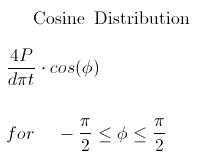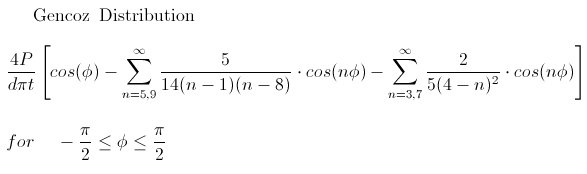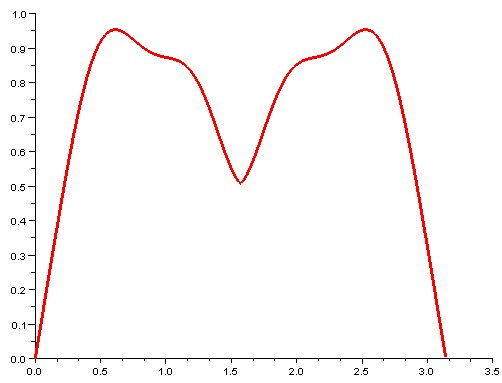## Bearing Loads: Cosine and Gencoz Distributions

A bearing load occurs at a hole which is loaded by the shank of a fastener or pin.  The pin applies a distributed load on the face of the hole.  Sinusoidal and parabolic distributions are commonly used to model the stress created by this interaction.  In addition, the Gencoz distribution is highly recommended.  The original study can be referenced in the paper Application of Finite Element Analysis Techniques for Predicting Crack Propagation in Lugs by O.Gencoz, U.G. Goranson and R.R. Merill from the International Journal of Fatigue in July 1980.  The book Practical Airframe Fatigue and Damage Tolerance is published by http://www.sigmak.ltd.uk and includes documentation of this distribution as well.  This book is highly recommended and filled to the brim with fascinating knowledge.  In addition the Roshaz FE software implements the Gencoz distribution and recommends its use in their online documentation.

When using this method assume a direction for the vector of applied force.  The pin applies a load about 180 degrees centered on the direction of the force vector.  Take the angles -90 to +90 from the direction of the applied vector for the functions below.  This causes a distribution of pressure about the face of the hole in bearing contact.  The magnitude of the applied pressure will be the a result of the following variables.

• P = applied force
• d = lug internal diameter
• t = lug thickness
• Φ = +/- angle from applied force vector

Note, the SciLab plots from below have been adjusted to plot the distribution about a hole size of radius of 1.  They have also been clocked such that they are symmetrical about the 90 degree mark in Scilab.  The magnitude of the 4P/(d*Π*t) term is set to 1 in the plots.The Gencoz Distribution is defined as a sum of terms.  This formulation was developed after careful examination of photoelastic models and correlation to FE code.  Note the index increment is four and starts and 5 and 3 respectively.Gencoz Distribution plotted in rectangular coordinates with a magnitude of 1.The following code segment is written in SciLab and originally based on Fortran code.  The Fortran code can be used directly and compiled with the freely available GNU gfortran compiler on Windows available through Cygwin or mingw-w64.  The Fortran code is shown commended out at the top of the segment followed by the SciLab function.

```//This scilab code is baised on the following Fortran function which was
//posted to solve for the Gencoz lug loading distribution of pressure
//from johnors at http://www.eng-tips.com/viewthread.cfm?qid=209705&page=9
//
//      FUNCTION GENCOZ(A)
//
//      IMPLICIT DOUBLE PRECISION (A-H,O-Z)
//
//      GENCOZ=COS(A)
//
//      IF (GENCOZ.LT.0.0D0) THEN
//         GENCOZ=0.0D0
//         RETURN
//      END IF
//
//      OLD=-1.0D0
//      J=1
//      K=-1
//   10 J=J+4
//      K=K+4
//      RJ=FLOAT(J)
//      RK=FLOAT(K)
//      GENCOZ=GENCOZ-COS(A*RJ)*5.0D0/((RJ-8.0D0)*(RJ-1.0D0)*14.0D0)
//     *             -COS(A*RK)*2.0D0/((RK-4.0D0)*(RK-4.0D0)*5.0D0)
//      IF (GENCOZ.LT.1.0D-8) RETURN
//      DIFF=ABS((GENCOZ-OLD)/OLD)
//      IF (DIFF.LT.1.0D-6) RETURN
//      OLD=GENCOZ
//      GO TO 10
//
//      END

function [gencozPres] = gencoz( angle )

gencozPres = cos(angle);

if( gencozPres < 0 )
gencozPres = 0;
return;
end

old = -1;
j = 1;
k = -1;

while( 1 )
j = j+4;
k = k+4;
gencozPres = gencozPres - ((cos(angle*j)*5) / ( (j-8)*(j-1)*14 ));
gencozPres = gencozPres - ((cos(angle*k)*2) / ( (k-4)*(k-4)*5 ));

if( gencozPres < (1*10^-8) )
break;
end
if( abs((gencozPres-old)/old) < (1*10^-6) )
break;
end

old = gencozPres;
end

endfunction //end gencoz```